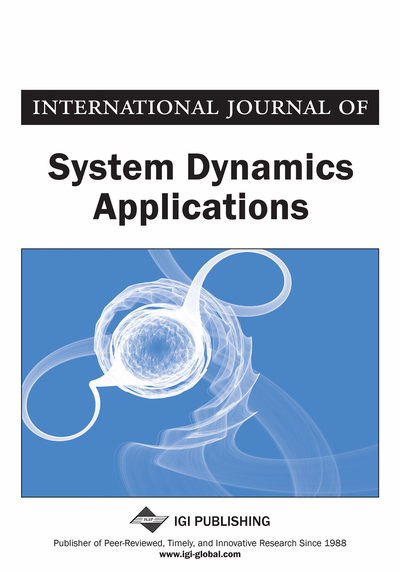# Stabilizing and Swinging-Up the Inverted Pendulum Using PI and PID Controllers Based on Reduced Linear Quadratic Regulator Tuned by PSO

M. E. Mousa (Ministry of Civil Aviation, Cairo, Egypt), M. A. Ebrahim (Electrical Engineering Department, Benha University, Cairo, Egypt) and M. A. Moustafa Hassan (Electrical Power Department, Cairo University, Giza, Egypt)
Copyright: © 2015 |Pages: 18
DOI: 10.4018/IJSDA.2015100104
OnDemand PDF Download:
Available
\$37.50
No Current Special Offers

## Abstract

The inherited instabilities in the Inverted Pendulum (IP) system make it one of the most difficult nonlinear problems in the control theory. In this research work, Proportional –Integral and Derivative (PID) Controller with a feed forward gain is used with Reduced Linear Quadratic Regulator (RLQR) for stabilizing the Cart Position and Swinging-up the Pendulum angle. Tuning the Controllers' gains is achieved by using Particle Swarm Optimization (PSO) Technique. Obtaining the combined PID controllers' gains with a feed forward gain and RLQR is a multi-dimensions control problem. The Proposed Controllers give minimum Settling Time, Rise Time, Undershoot and Over shoot for both the Cart Position and the Pendulum angle. A disturbance with different amplitudes is applied to the system, and the results showed the robustness of the systems based on the tuned controllers. The overall results are promising.
Article Preview
Top

## 1. Introduction

Inverted pendulum system is unstable, nonlinear, non-minimum phase and under actuated system. It’s a classical benchmark for design and comparing of different control techniques whatever it is conventional or modern. The concept of the Inverted Pendulum System is used in many modern technology applications like landing of aircrafts, space satellites, launching and guidance of the missile operations, spacecraft, statistics applications, and biomechanics (Chakraborty, 2014). Intrinsic nonlinearity of Inverted pendulum system make it subject of an interesting from the stand point of control (Mills, et al., 2009). The evaluation of various control theories is based on the inverted pendulum system (Wu, et al., 2015). Inverted pendulum system is a physical system consists of bar which is usually made of aluminum and swinging around the fixed pivot. This fixed pivot will be installed on the vehicle which moves in the horizontal direction only. The center of gravity of the normal Pendulum is under the axis of rotation and therefore, it's condition is stable when it is directed to the bottom while the center of gravity of the inverted pendulum is over its axis of rotation. In the inverted pendulum problem, the pendulum tried to be in a vertical position to be heading up. The swinging up of the pendulum makes the situation of the system abnormal. So, permanent controller should be applied to the system to keep the pendulum vertically upright. Fast swinging up of the pendulum angle and stabilizing the cart at a certain position are required. Linear inverted pendulum system and rotary inverted pendulum system are the most common types of inverted pendulum systems. Each type of the inverted pendulum systems has its dynamic modeling. In this paper, linear inverted pendulum system is considered. It may be called cart Inverted pendulum system as it has a cart which carries the pendulum.

The Inverted pendulum system has its mass above its pivot point which the cart carried the pendulum. It is inherently unstable system so the applied controllers need to apply permanently. Cart inverted pendulum system has one input and two outputs. The input is the force that applied to the cart. The two outputs are the cart position and the pendulum angle. It makes the system single input multi output system (SIMO). The main task for the controller is stabilizing the cart position and swinging up the pendulum angle.

In order to stabilize the inverted pendulum, many researches proposed varieties of control strategies such as PID control strategy, Sliding mode control strategy (Azar & Serrano, 2015a), Linear Quadratic Regulator Control Strategy, Fuzzy Logic Control strategy, Fractional PID control strategy and Artificial methods (Azar & Vaidyanathan, 2015; Zhu Q & Azar, 2015; Azar & Serrano, 2015c).

PID controller is used widely in different control applications (Azar & Serrano, 2014; Azar & Serrano, 2015b). It is the most common controller used as feedback controller. It calculates the error value which defined as the difference between the measured value and the desired value. By adjusting the controller gain, the error is minimized to enhance the performance of the system. Rise time, settling time, overshoot and undershoot are also improved. Linear Quadratic Regulator (LQR) is used to design linear controller for linear systems. It depends on solving the Riccati equations then the LQR gain can be obtained. Solving Riccati equation is not an easy task because it has two designing matrices Q and R. It must be positive definite and positive semi-definite respectively. Designing the LQR gain is mainly depending on these two matrices. Computational intelligent techniques can be used to tune the controllers' parameters. PSO is one of the most commonly used computational evolutionary intelligence techniques that used in control theory (Azar & Vaidyanathan, 2014). The concept of the evolutionary systems is used a population of candidate solutions to the problem based on inspired natural selection operators. “Evolution strategy” is presented by Rechenberg and used it to optimize real−valued parameters for devices such as airfoils. Then the research is grown in evolution strategy area.

## Complete Article List

Search this Journal:
Reset
Volume 11: 2 Issues (2022): 1 Released, 1 Forthcoming
Volume 10: 4 Issues (2021)
Volume 9: 4 Issues (2020)
Volume 8: 4 Issues (2019)
Volume 7: 4 Issues (2018)
Volume 6: 4 Issues (2017)
Volume 5: 4 Issues (2016)
Volume 4: 4 Issues (2015)
Volume 3: 4 Issues (2014)
Volume 2: 4 Issues (2013)
Volume 1: 4 Issues (2012)
View Complete Journal Contents Listing